StatLect

# Logistic classification model (logit or logistic regression)

The logistic classification model (or logit model) is a binary classification model in which the conditional probability of one of the two possible realizations of the output variable is assumed to be equal to a linear combination of the input variables, transformed by the logistic function.## Classification vs regression

A logit model is often called logistic regression model. However, in these lecture notes we prefer to stick to the convention (widespread in the machine learning community) of using the term regression only for conditional models in which the output variable is continuous. So we use the term classification here because in a logit model the output is discrete.

## Model specification

Suppose that we observe a sample of datafor. Each observation in the sample is made up of:

• an output variable denoted by;

• avector of inputs, denoted by.

It is assumed that the outputcan take only two values, either 1 or 0 (it is a Bernoulli random variable).

The probability that the outputis equal to 1, conditional on the inputs, is assumed to bewhereis the logistic function andis avector of coefficients.

It is immediate to see that the logistic functionis always positive. Furthermore, it is increasing andso that it satisfiesThus,is a well-defined probability because it lies between 0 and 1.

Since probabilities need to sum up to 1, the probability that the outputis equal to 0 (the only other possible realization of) is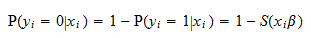## Explanation

Why is the logistic classification model specified in this manner? Why is the logistic function used to transform the linear combination of inputs?

The simple answer is that we would like to do something similar to what we do in a linear regression model: use a linear combination of the inputs as our prediction of the output. However, our prediction needs to be a probability and there is no guarantee that the linear combinationis between 0 and 1. Thus, we use the logistic function because it provides a convenient way of transformingand forcing it to lie in the interval between 0 and 1.

We could have used other functions that enjoy properties similar to the logistic function. As a matter of fact, other popular classification models can be obtained by simply substituting the logistic function with another function and leaving everything else in the model unchanged. For example, by substituting the logit function with the cumulative distribution function of a standard normal distribution, we obtain the so-called probit model.

## The logit model as a latent variable model

Another way of thinking about the logit model is to define a latent variable (i.e., an unobserved variable)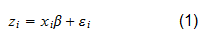whereis a random error term that adds noise to the relationship between the inputsand the variable. The latent variableis then assumed to determine the outputas follows: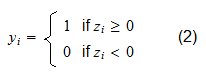From these assumptions and the additional assumption thathas a symmetric distribution aroundit follows that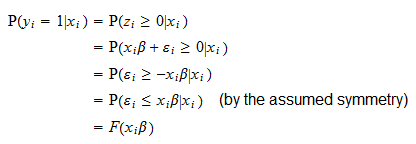whereis the cumulative distribution function of the error.

It turns out that the logistic function used to define the logit model is the cumulative distribution function of a symmetric probability distribution called standard logistic distribution. Therefore, the logit model can be written as a latent variable model, specified by equations (1) and (2) above, in which the errorhas a logistic distribution.

By choosing different distributions for the error, we obtain other binary classification models. For example, if we assume thathas a standard normal distribution, then we obtain the so-called probit model.

## Estimation by maximum likelihood

The vector of coefficientsis often estimated by maximum likelihood methods.

Assume that the observationsin the sample are IID and denote thevector of all outputs byand thematrix of all inputs by. The latter is assumed to have full rank.

It is possible to prove (see the lecture on Maximum likelihood estimation of the logit model) that the maximum likelihood estimator(when it exists) can be obtained by performing simple Newton-Raphson iterations as follows:

• start from a guess(e.g.,);

• recursively update the guess: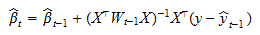where:andis andiagonal matrix (i.e., having all off-diagonal elements equal to) such that the elements on its diagonal are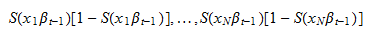• stop when numerical convergence is achieved, that is, when the difference betweenandis so small as to be negligible;

• set the maximum likelihood estimatorequal to the last update(denote the last iteration by).

The asymptotic covariance matrix of the maximum likelihood estimatorcan be consistently estimated by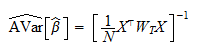so that the distribution of the estimatoris approximately normal with mean equal toand covariance matrix.

## Hypothesis testing

If the logit model is estimated with the maximum likelihood procedure illustrated above, any one of the classical tests based on maximum likelihood procedures (e.g., Wald, Likelihood Ratio, Lagrange Multiplier) can be used to test an hypothesis about the vector of coefficients.

Other tests can be constructed by exploiting the asymptotic normality of the maximum likelihood estimator. For example, we can perform a z test to test the null hypothesiswhereis the-th entry of the vector of coefficientsand.

The test statistic iswhereis the-th entry ofandis the-th entry on the diagonal of the matrix.

As the sample sizeincreases,converges in distribution to a standard normal distribution. The latter distribution can be used to derive critical values and perform the test.

Proof

We haveBy the asymptotic normality of the maximum likelihood estimator, the numeratorconverges in distribution to a normal random variable with mean. Furthermore, the consistency of our estimator of the asymptotic covariance matrix implies that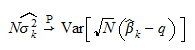wheredenotes convergence in probability. By the Continuous Mapping theorem,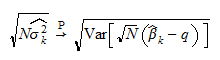and, by Slutsky's theorem,converges in distribution to a standard normal random variable.

The book

Most of the learning materials found on this website are now available in a traditional textbook format.

Glossary entries
Share